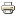On the manipulability of competitive equilibrium rules in many-to-many buyer–seller marketsArticle
Author/s:
David Pérez-Castrillo, Marilda Sotomayor
International Journal of Game Theory
Publisher:
Springer
Year:
2017
Journal pages:
1-25
We analyze the manipulability of competitive equilibrium allocation rules for the simplest many-to-many extension of Shapley and Shubik’s (Int J Game Theory 1:111–130, 1972) assignment game. First, we show that if an agent has a quota of one, then she does not have an incentive to manipulate any competitive equilibrium rule that gives her her most preferred competitive equilibrium payoff when she reports truthfully. In particular, this result extends to the one-to-many (respectively, many-to-one) models the Non-Manipulability Theorem of the buyers (respectively, sellers), proven by Demange (Strategyproofness in the assignment market game. École Polytechnique, Laboratoire d’Économetrie, Paris, 1982), Leonard (J Polit Econ 91:461–479, 1983), and Demange and Gale (Econometrica 55:873–888, 1985) for the assignment game. Second, we prove a “General Manipulability Theorem” that implies and generalizes two “folk theorems” for the assignment game, the Manipulability Theorem and the General Impossibility Theorem, never proven before. For the one-to-one case, this result provides a sort of converse of the Non-Manipulability Theorem.
Tags:
Developed by Paolo Gittoi